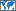## MATLAB

 Definition : Matrix Laboratory Category : Computing » Programming & Development Country/Region : WorldwidePopularity :

# What does MATLAB mean?

MATLAB (Matrix Laboratory) is a programming environment for algorithm development, data analysis, visualization, and numerical computation, developed by MathWorks. MATLAB is widely used for for matrix-based computation designed for scientific and engineering use. It is also good for many forms of numeric computation and visualization. MATLAB can be used in wide range of applications, including signal and image processing, communications, control design, test and measurement, financial modeling and analysis, computational biology etc.